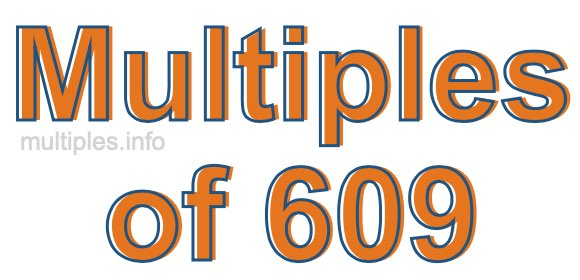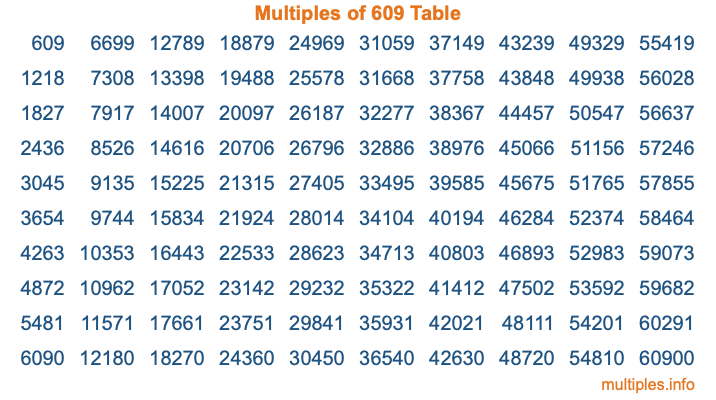Multiples of 609Welcome to the Multiples of 609 page. Here we will first teach you everything you will ever need to know about the multiples of 609, and then give you a study guide summary of everything we taught you to make sure you remember it all. Use this page to look up facts and learn information about the multiples of 609. This page will make you a multiples of six hundred nine expert!

Definition of Multiples of 609
Multiples of 609 are all the numbers that when divided by 609 equal an integer. Each of the multiples of 609 are called a multiple. A multiple of 609 is created by multiplying 609 by an integer.

Therefore, to create a list of multiples of 609, you start with 1 multiplied by 609, then 2 multiplied by 609, then 3 multiplied by 609, and so on for as long as you want. Thus, the list of the first five multiples of 609 is 609, 1218, 1827, 2436, and 3045. To see a larger list of multiples of 609, see the printable image of Multiples of 609 further down on this page. We also have a category where you can choose any nth multiple of 609.

Multiples of 609 Checker
The Multiples of 609 Checker below checks to see if any number of your choice is a multiple of 609. In other words, it checks to see if there is any number (integer) that when multiplied by 609 will equal your number. To do that, we divide your number by 609. If the the quotient is an integer, then your number is a multiple of 609.

Is  a multiple of 609?

Least Common Multiple of 609 and ...
A Least Common Multiple (LCM) is the lowest multiple that two or more numbers have in common. This is also called the smallest common multiple or lowest common multiple and is useful to know when you are adding our subtracting fractions. Enter one or more numbers below (609 is already entered) to find the LCM.

Check out our LCM Calculator if you need more details about the Least Common Multiple or if you need the LCM for different numbers for adding and subtraction fractions.

nth Multiple of 609
As we stated above, 609 is the first multiple of 609, 1218 is the second multiple of 609, 1827 is the third multiple of 609, and so on. Enter a number below to find the nth multiple of 609.

th multiple of 609

Multiples of 609 vs Factors of 609
609 is a multiple of 609 and a factor of 609, but that is where the similarities end. All postive multiples of 609 are 609 or greater than 609. All positive factors of 609 are 609 or less than 609.

Below is the beginning list of multiples of 609 and the factors of 609 so you can compare:

Multiples of 609: 609, 1218, 1827, 2436, 3045, etc.

Factors of 609: 1, 3, 7, 21, 29, 87, 203, 609

As you can see, the multiples of 609 are all the numbers that you can divide by 609 to get a whole number. The factors of 609, on the other hand, are all the whole numbers that you can multiply by another whole number to get 609.

It's also interesting to note that if a number (x) is a factor of 609, then 609 will also be a multiple of that number (x).

Multiples of 609 vs Divisors of 609
The divisors of 609 are all the integers that 609 can be divided by evenly. Below is a list of the divisors of 609.

Divisors of 609: 1, 3, 7, 21, 29, 87, 203, 609

The interesting thing to note here is that if you take any multiple of 609 and divide it by a divisor of 609, you will see that the quotient is an integer.

Multiples of 609 Table
Below is an image of the first 100 multiples of 609 in a table. The table is in chronological order, column by column. The first column has the first ten multiples of 609, the second column has the next ten multiples of 609, and so on.The Multiples of 609 Table is also referred to as the 609 Times Table or Times Table of 609. You are welcome to print out our table for your studies.

Negative Multiples of 609
Although not often discussed or needed in math, it is worth mentioning that you can make a list of negative multiples of 609 by multiplying 609 by -1, then by -2, then by -3, and so on, to get the following list of negative multiples of 609:

-609, -1218, -1827, -2436, -3045, etc.

Multiples of 609 Summary
Below is a summary of important Multiples of 609 facts that we have discussed on this page. To retain the knowledge on this page, we recommend that you read through the summary and explain to yourself or a study partner why they hold true.

There are an infinite number of multiples of 609.

A multiple of 609 divided by 609 will equal a whole number.

609 divided by a factor of 609 equals a divisor of 609.

The nth multiple of 609 is n times 609.

The largest factor of 609 is equal to the first positive multiple of 609.

609 is a multiple of every factor of 609.

609 is a multiple of 609.

A multiple of 609 divided by a divisor of 609 equals an integer.

609 divided by a divisor of 609 equals a factor of 609.

Any integer times 609 will equal a multiple of 609.

Multiples of a Number
Here you can get the multiples of another number, all with the same attention to detail as we did for multiples of 609 on this page.

Multiples of
Multiples of 610
Did you find our page about multiples of six hundred nine educational? Do you want more knowledge? Check out the multiples of the next number on our list!